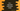# C++ program to calculate arithmetic mean# How to calculate arithmetic mean in C++ :

Arithmetic mean is equal to the average of numbers. It is equal to the sum of numbers divide by the count of numbers. For example, the arithmetic mean of n1,n2 and n3 is (n1 + n2 + n3)/3.

In this post, we will learn how to calculate the arithmetic mean for user input numbers.

The program will ask the user to enter the count of numbers, it will then take these numbers as input from the user and calculate the arithmetic mean.

## C++ program :

The C++ program to calculate arithmetic mean is as below :

``````#include <iostream>
using namespace std;

int main()
{
//1
int total;
int sum = 0;
int currentNumber;

//2
cout << "Enter total count : " << endl; cin >> total;

//3
for (int i = 0; i < total; i++)
{
//4
cout << "Enter number " << i + 1 << " :" << endl; cin >> currentNumber;
sum += currentNumber;
}

//5
cout << "Arithmetic Mean : " << (sum / total) << endl;

return 0;
}``````

### Explanation :

The commented numbers in the above program denote the step numbers below :

1. Create three variables total, sum and currentNumber to hold the total count, sum of all numbers and current user input number.
2. Ask the user to enter the total count of numbers. Read it and store it in the variable total.
3. Run one for loop to read the numbers one by one.
4. Inside the loop, ask the user to enter a number, read it using cin and store it temporarily in currentNumber. Also, add currentNumber to sum.
5. Finally, print out the arithmetic mean. Arithmetic mean is equal to sum/total.

### Sample Output :

``````Enter total count :
5
Enter number 1 :
2
Enter number 2 :
4
Enter number 3 :
6
Enter number 4 :
8
Enter number 5 :
10
Arithmetic Mean : 6``````